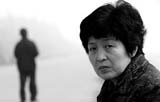# CCTV：2009感动中国候选人物网络投票2009，谁最让您感动？

网络投票截止日期：2010年1月24日00:00

感动中国推选委员会推荐候选人

1、陈玉蓉2、何东旭、陈及时、方招：救人牺牲的三个大学生   <<<< 详细事迹

3、朱邦月  照顾残疾家人20多年的乐观老人    <<<< 详细事迹

4、王素贤  照顾残疾妹妹半个世纪    <<<< 详细事迹

5、吕正操  开国上将    <<<< 详细事迹

6、潘美儿  南丁格尔奖获得者    <<<< 详细事迹

7、周火生  把一生经历都献给学生的希望老人    <<<< 详细事迹

8、任菲莉  照顾残疾女儿22年，并创造奇迹    <<<< 详细事迹

9、卓 琳   把个人的积蓄10万元全部捐给地震灾区。    <<<< 详细事迹

10、宋文骢  歼10 设计师    <<<< 详细事迹

11、李  灵  为留守儿童办学    <<<< 详细事迹

12、尼  帕  多民族孤儿的妈妈    <<<< 详细事迹

13、苏珊·坦芭肯  给高原的盲童带来光明    <<<< 详细事迹

14、阳早 寒春  共和国的好朋友    <<<< 详细事迹

15、佘太和  好干部    <<<< 详细事迹

16、黄秀英夫妇 用车拉出少儿学校    <<<< 详细事迹

17、张正祥  26年滇池保卫战    <<<< 详细事迹

18、次仁曲珍  坚持40年每天升国旗的藏族老人    <<<< 详细事迹

19、三位矿工  604小时创造矿难史奇迹    <<<< 详细事迹

20、王艾甫 　送烈士魂归故里    <<<< 详细事迹

21、翟墨     中国人首次单人无动力帆船环球航海  <<<< 详细事迹

22、张仁杰  《感恩中国》公益网站制作人    <<<< 详细事迹

23、程  明   累死在打黑一线    <<<< 详细事迹

24、滕大韶  乡村小学教师    <<<< 详细事迹

25、豆洪波  被爱心救助的希望小学老师    <<<< 详细事迹

26、万金刚  牺牲在抗暴一线的武警战士    <<<< 详细事迹

27、梁思礼   火箭专家    <<<< 详细事迹

28、张曙光   中国火车提速设计师    <<<<详细事迹

29、何小川   带村民致富的好村长    <<<< 详细事迹

30、赵  鹏   “80后”山村教师感动八方 <<<< 详细事迹

31、徐兆华    一级英模本色不改好片警      <<<< 详细事迹

32、沈浩      人民的好村官                <<<< 详细事迹